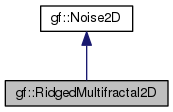Gamedev Framework (gf)  0.9.0 A C++14 framework for 2D games
gf::RidgedMultifractal2D Class Reference

Ridged Multifractal 2D noise. More...

#include <gf/Noises.h>

Inheritance diagram for gf::RidgedMultifractal2D:[legend]

Public Member Functions

RidgedMultifractal2D (Noise2D &noise, double scale, double offset=1.0, double gain=1.0, std::size_t octaves=8, double lacunarity=2.0, double persistence=0.5, double dimension=1.0)
Constructor. More...

virtual double getValue (double x, double y) override
Take a 2D noise value. More...Public Member Functions inherited from gf::Noise2D
virtual ~Noise2D ()
Virtual destructor. More...

double operator() (double x, double y)
Take a 2D noise value. More...

Detailed Description

Ridged Multifractal 2D noise.

◆ RidgedMultifractal2D()

 gf::RidgedMultifractal2D::RidgedMultifractal2D ( Noise2D & noise, double scale, double offset = 1.0, double gain = 1.0, std::size_t octaves = 8, double lacunarity = 2.0, double persistence = 0.5, double dimension = 1.0 )

Constructor.

Parameters
 noise The basic noise function scale The scale factor offset The offset gain The gain octaves The number of octaves lacunarity The factor applied to frequency persistence The factor applied to amplitude dimension The contrast between the layers

◆ getValue()

 virtual double gf::RidgedMultifractal2D::getValue ( double x, double y )
overridevirtual

Take a 2D noise value.

Parameters
 x The x coordinate of the noise value y The y coordinate of the noise value
Returns
The noise value

Implements gf::Noise2D.